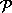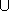## CGAL::surface_neighbors_3

### Definition

Given a set of sample points issued from a surface and a query point p, the function surface_neighbors_3 computes the neighbors of p on the surface within the sample points. If the sampling is sufficiently dense, the neighbors are provably close to the point p on the surface (cf. the manual pages and [BF02],[Flö03]). They are defined to be the neighbors of p in the regular triangulation dual to the power diagram which is equivalent to the intersection of the Voronoi cell of the query point p with the tangent plane to the surface at p.

#include <CGAL/surface_neighbors_3.h>

template <class OutputIterator, class InputIterator, class Kernel>
OutputIterator
 surface_neighbors_3 ( InputIterator first, InputIterator beyond, typename Kernel::Point_3 p, typename Kernel::Vector_3 normal, OutputIterator out, Kernel K)
The sample pointsare provided in the range [.first, beyond.). InputIterator::value_type is the point type Kernel::Point_3. The tangent plane is defined by the point p and the vector normal. The parameter K determines the kernel type that will instantiate the template parameter of Voronoi_intersection_2_traits_3<K>.
The surface neighbors of p are computed which are the neighbors of p in the regular triangulation that is dual to the intersection of the 3D Voronoi diagram ofwith the tangent plane. The point sequence that is computed by the function is placed starting at out. The function returns an iterator that is placed past-the-end of the resulting point sequence.

template <class OutputIterator, class InputIterator, class ITraits>
OutputIterator
 surface_neighbors_3 ( InputIterator first, InputIterator beyond, typename ITraits::Point_2 p, OutputIterator out, ITraits traits)
the same as above only that the traits class must be instantiated by the user. ITraits must be equivalent to Voronoi_intersection_2_traits_3<K>.

The next functions return, in addition, a boolean value that certifies whether or not, the Voronoi cell of p can be affected by points that lie outside the input range, i.e. outside the ball centered on p passing through the furthest sample point from p in the range [.first, beyond.). If the sample points are collected by a k-nearest neighbor or a range search query, this permits to verify that a large enough neighborhood has been considered.

template <class OutputIterator, class InputIterator, class Kernel>
std::pair< OutputIterator, bool >
 surface_neighbors_certified_3 ( InputIterator first, InputIterator beyond, typename Kernel::Point_3 p, typename Kernel::Vector_3 normal, OutputIterator out, Kernel K)
Similar to the first function. The additional third return value is true if the furthest point in the range [.first, beyond.) is further away from p than twice the distance from p to the furthest vertex of the intersection of the Voronoi cell of p with the tangent plane defined be (p,normal). It is false otherwise.

template <class OutputIterator, class InputIterator, class Kernel>
std::pair< OutputIterator, bool >
 surface_neighbors_certified_3 ( InputIterator first, InputIterator beyond, typename Kernel::Point_2 p, typename Kernel::FT max_distance, OutputIterator out, Kernel kernel)
The same as above except that this function takes the maximal distance from p to the points in the range [.first, beyond.) as additional parameter.

template <class OutputIterator, class InputIterator, class ITraits>
std::pair< OutputIterator, bool >
 surface_neighbors_certified_3 ( InputIterator first, InputIterator beyond, typename ITraits::Point_2 p, OutputIterator out, ITraits traits)
The same as above only that the traits class must be instantiated by the user. ITraits must be equivalent to Voronoi_intersection_2_traits_3<K>. There is no parameter max_distance.

template <class OutputIterator, class InputIterator, class ITraits>
std::pair< OutputIterator, bool >
 surface_neighbors_certified_3 ( InputIterator first, InputIterator beyond, typename ITraits::Point_2 p, typename ITraits::FT max_distance, OutputIterator out, ITraits traits)
The same as above with the parameter max_distance.

The next function allows to filter some potential neighbors of the query point p fromvia its three-dimensional Delaunay triangulation. All surface neighbors of p are necessarily neighbors in the Delaunay triangulation of{p}.

template < class Dt, class OutputIterator >
OutputIterator
 surface_neighbors_3 ( Dt dt, typename Dt::Geom_traits::Point_2 p, typename Dt::Geom_traits::Vector_3 normal, OutputIterator out, typename Dt::Face_handle start = typename Dt::Face_handle())
computes the surface neighbor coordinates with respect to the points that are vertices of the Delaunay triangulation dt. The type Dt must be equivalent to Delaunay_triangulation_3<Gt, Tds>. The optional parameter start is used for the used as a starting place for the search of the conflict zone. It may be the result of the call dt.locate(p). This function instantiates the template parameter ITraits to be Voronoi_intersection_2_traits_3<Dt::Geom_traits>.

template < class Dt, class OutputIterator, class ITraits>
OutputIterator
 surface_neighbors_3 ( Dt dt, typename Dt::Geom_traits::Point_2 p, OutputIterator out, ITraits traits, typename Dt::Face_handle start = typename Dt::Face_handle())
The same as above only that the parameter traits instantiates the geometric traits class. Its type ITraits must be equivalent to Voronoi_intersection_2_traits_3<K>.

### Requirements

1. Dt is equivalent to the class Delaunay_triangulation_3.
2. OutputIterator::value_type is equivalent to Dt::Point_3, i.e. a point type.
3. ITraits is equivalent to the class Voronoi_intersection_2_traits_3<K>.